Next: Bibliography Up: Dissertation Hajdin Ceric Previous: A. Vacancies in Solids

# B. Some Tools from Abstract Mathematical Analysis

Let V be a Hilbert space andand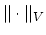, the corresponding scalar product and norm, respectively. A linear form (or linear functional)on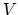is a function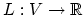such that,(B.1)

A linear formis bounded if there is a constant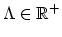such that,(B.2)

A bilinear form onis a function, which is linear in each argument separately, i.e., such that, for all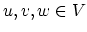and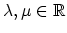,(B.3)(B.4)

The bilinear formis said to be symmetric if,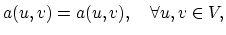(B.5)

bounded if there is a constantsuch that,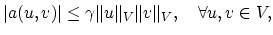(B.6)

and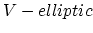if there is a constant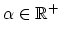such that,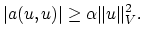(B.7)

The set of all bounded linear functionals onis called dual space ofand denoted. The norm inis given by,(B.8)

Theorem I (Riesz's representation theorem): Letbe a Hilbert space with scalar product. For each bounded linear functionalonthere is an uniquesuch that,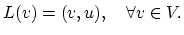(B.9)

Moreover,(B.10)

Theorem II (Lax-Milgram lemma): If the bilinear formis bounded and-elliptic in the Hilbert space, andis bounded linear form in, than there exists a unique vectorsuch that,(B.11)

and,(B.12)

Theorem III: Assume thatis a symmetric,-elliptic bilinear form and thatis a bounded linear form on the Hilbert space. Thansatisfies (B.11) if and only if,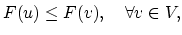where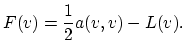(B.13)Next: Bibliography Up: Dissertation Hajdin Ceric Previous: A. Vacancies in Solids

H. Ceric: Numerical Techniques in Modern TCAD